Function Repository Resource:

# SIConstantConvert

Transform a unit into a product of constants

Contributed by: Peter Cullen Burbery
 ResourceFunction["SIConstantConvert"][unit] transforms unit into a product of the seven SI defining constants. ResourceFunction["SIConstantConvert"][quantitydistribution] transforms quantitydistribution into a quantity distribution with units that are the product of the seven SI defining constants.

## Details

The defining constants are available in the BIPM’s SI Brochure and NIST’s SI Brochure.
Defining the SI base units in terms of physical constants enables realizing the units with an accuracy that is limited only by the quantum structure of nature and our technical abilities but not by the definitions themselves.
The seven defining constants for the second, meter, kilogram, ampere, kelvin, mole, and candela are the hyperfine transition frequency of cesium-133, the speed of light in vacuum, the Planck constant, the Boltzmann constant, the Avogadro constant, and the the luminous efficacy of monochromatic radiation of frequency 540 × 1012 hertz, respectively.

## Examples

### Basic Examples (3)

Convert the height of the Empire State building to a combination of physical constants:

 In:=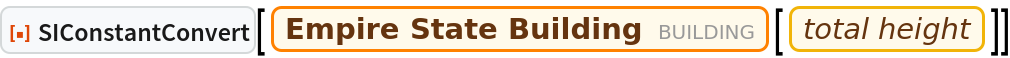Out=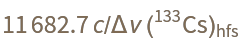Transform the seven SI base units into products of constants:

 In:=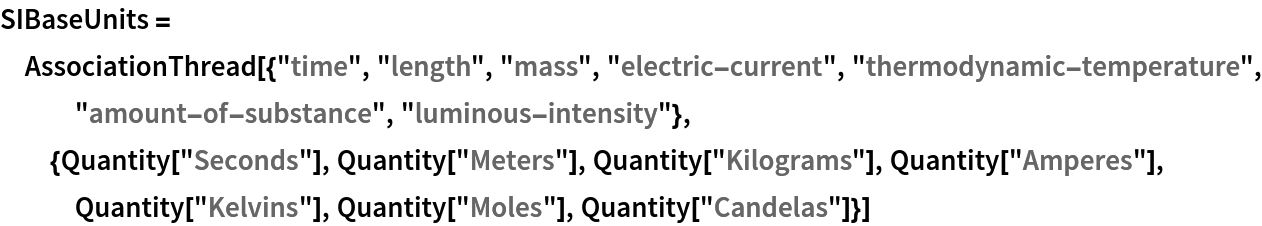Out=In:=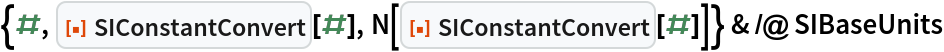Out=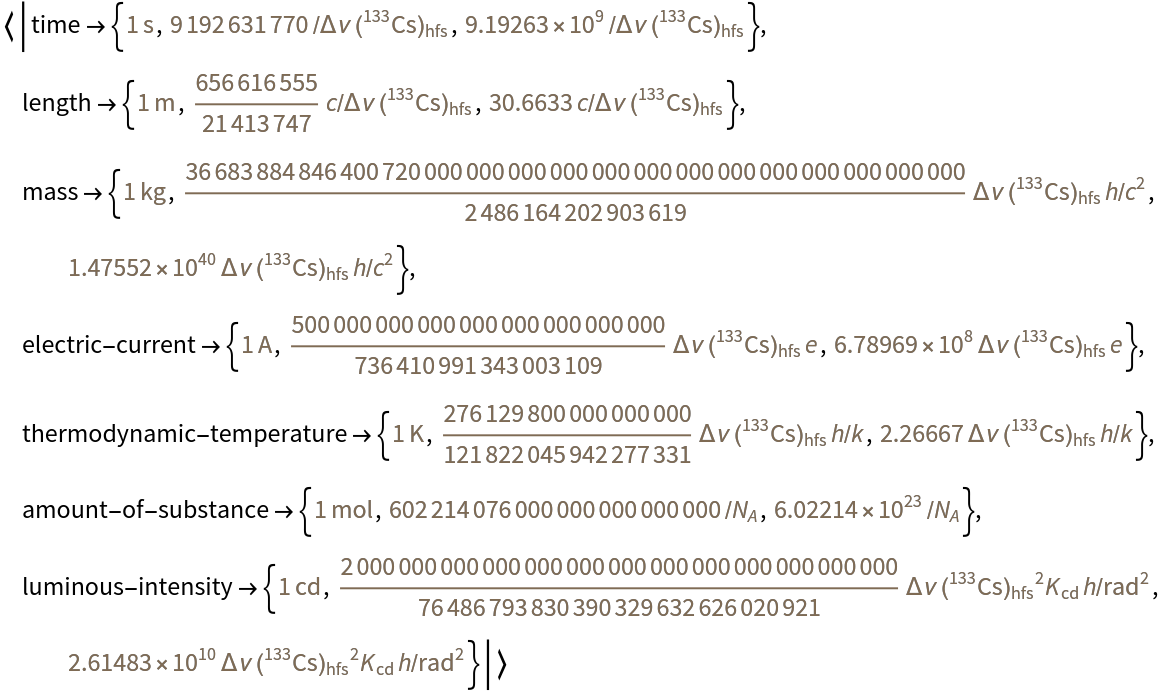Fit a model to data with units:

 In:=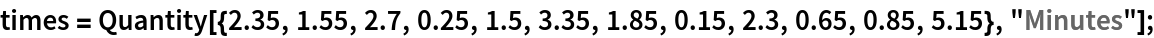Convert the distribution to a form with the SI constants:

 In:=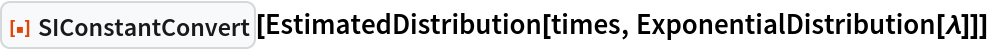Out=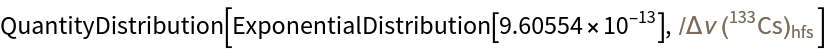### Applications (4)

Transform the 22 SI derived units with special names to products of constants:

 In:=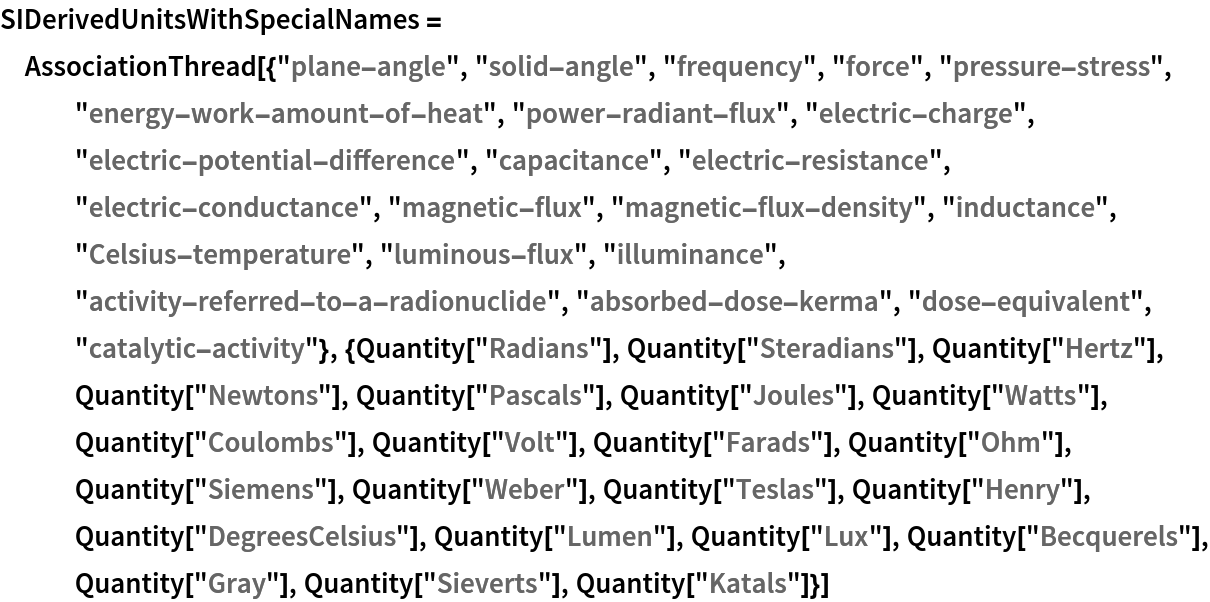Out=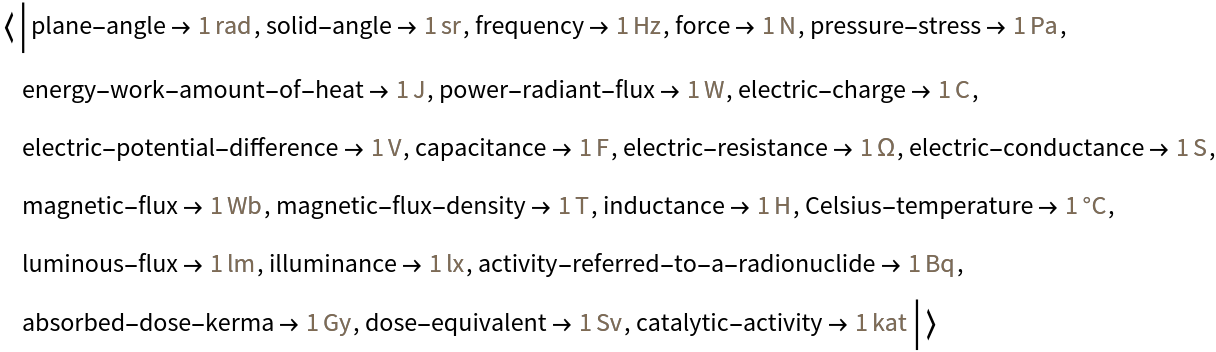In:=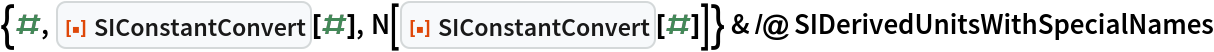Out=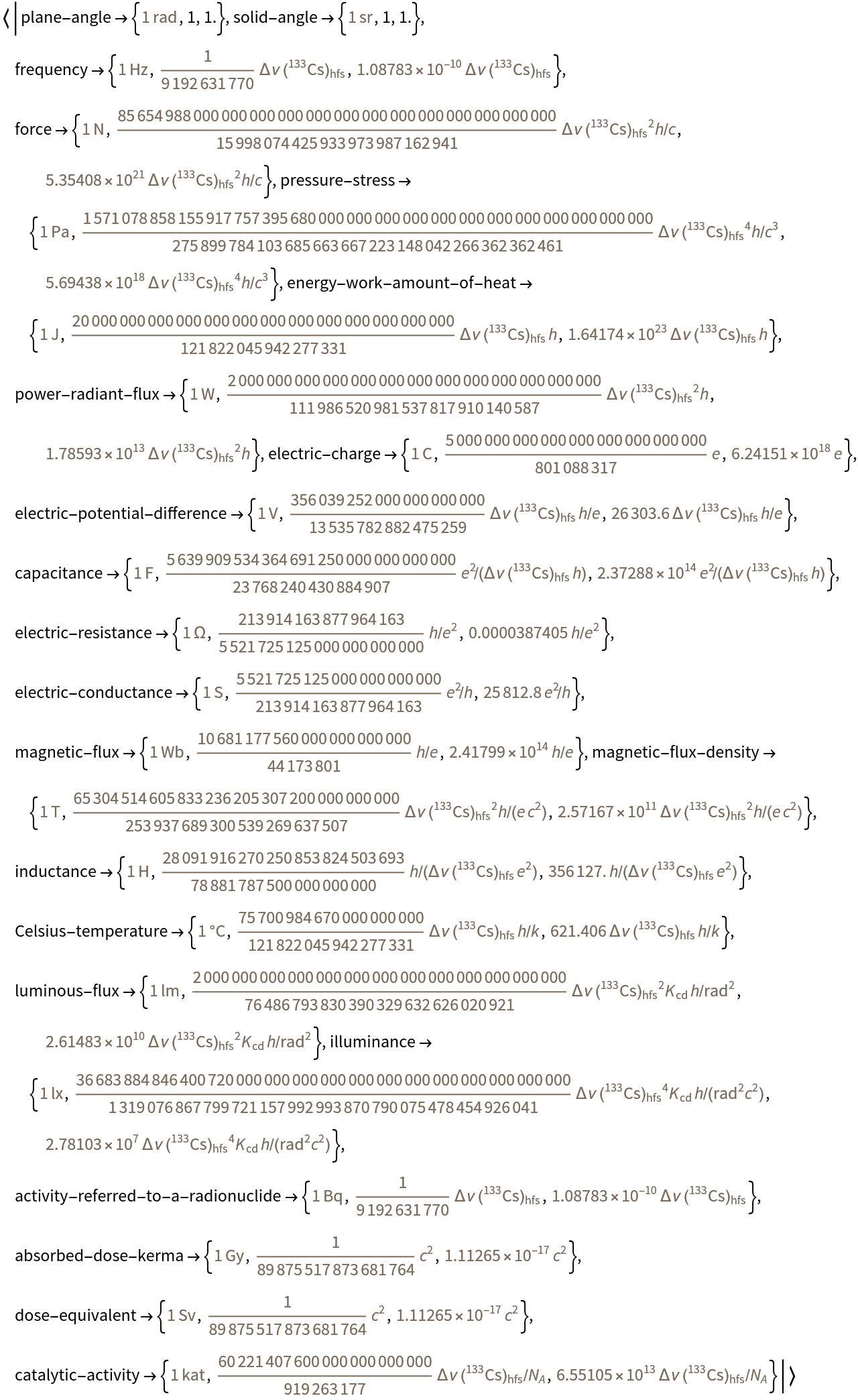Transform physical quantities expressed in derived units with only the seven base units to products of constants:

 In:=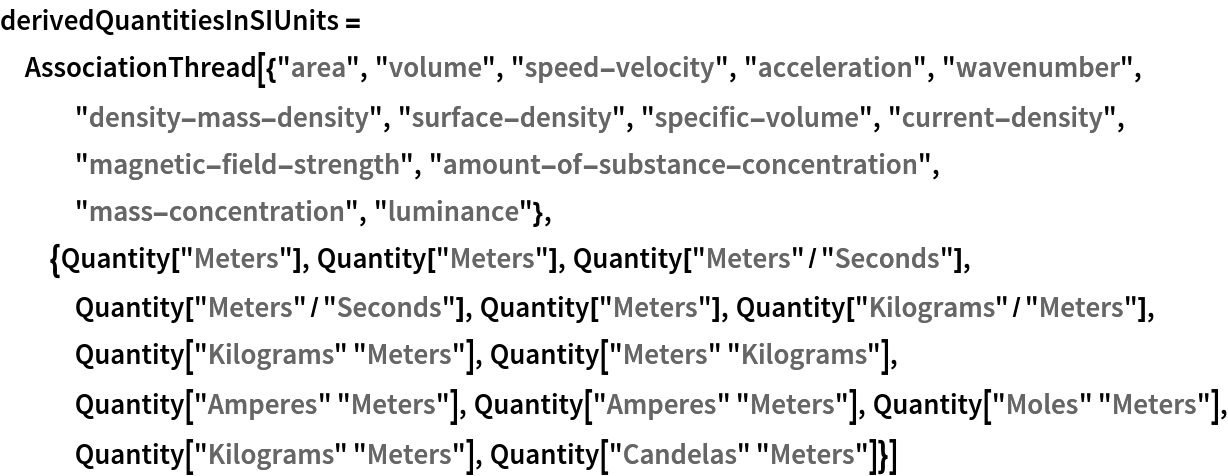Out=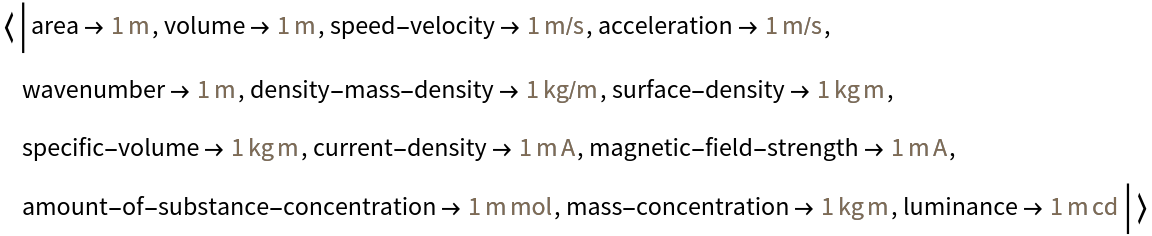In:=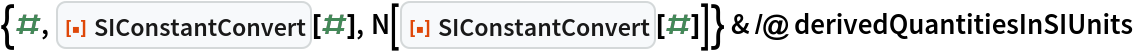Out=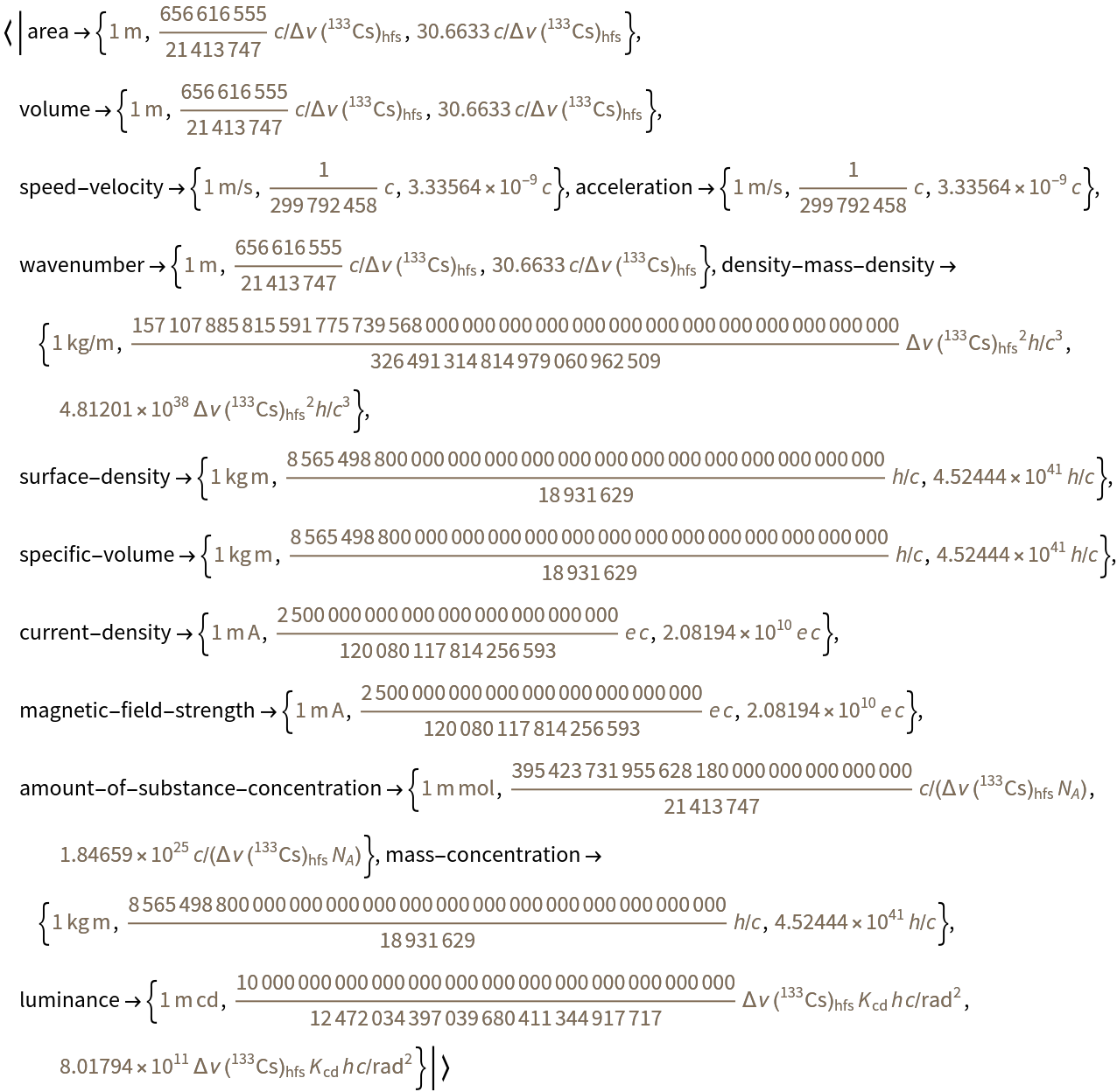Transform SI coherent derived units whose names and symbols include coherent derived units with special names and symbols to products of constants:

 In:=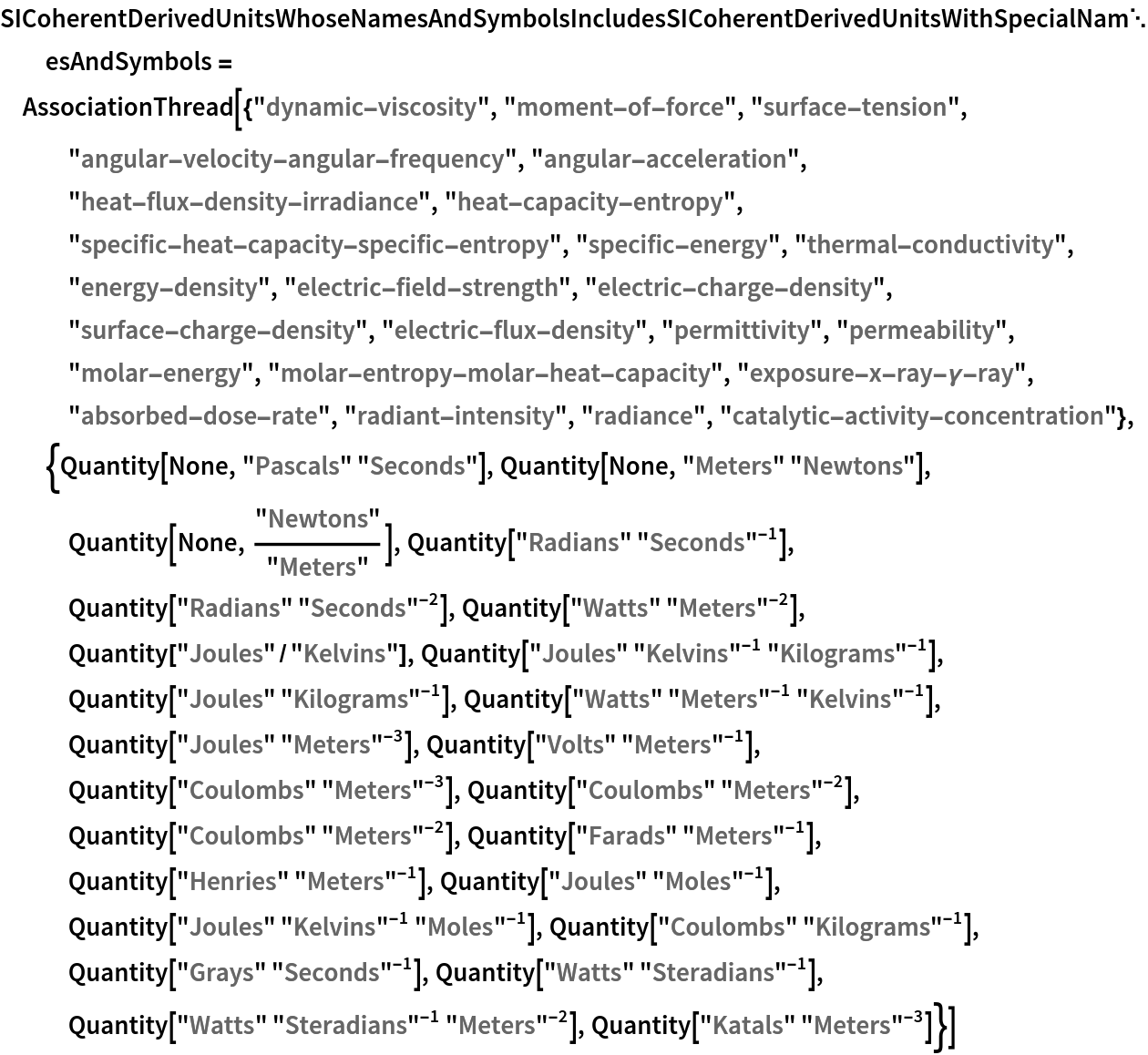Out=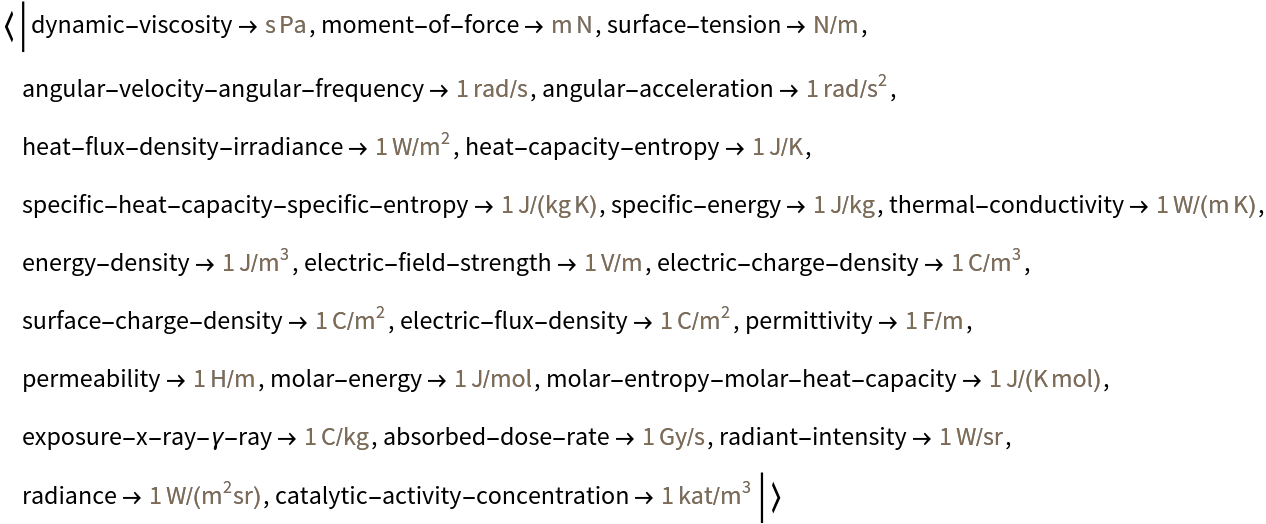In:=Out=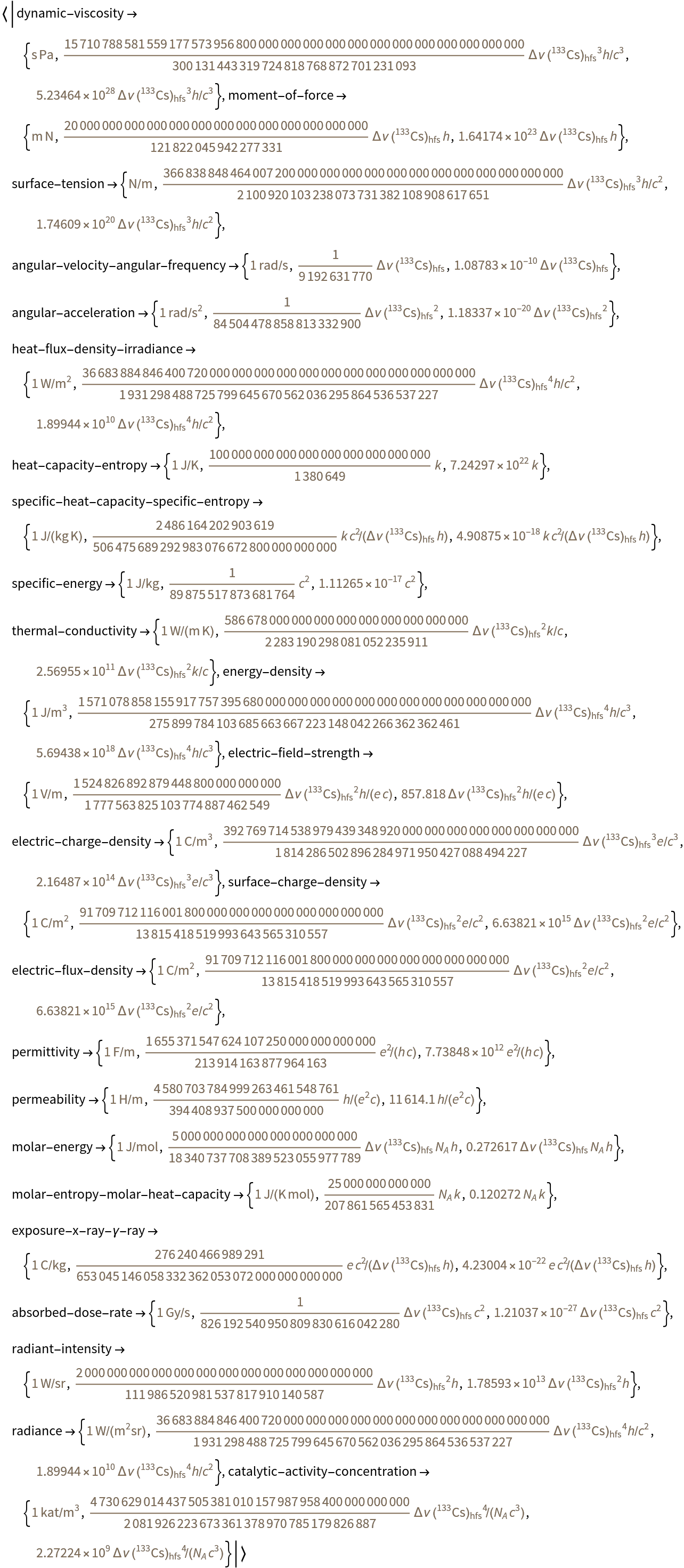Estimate the distribution of the daily mean temperature in Chicago in the summer of 2015:

 In:=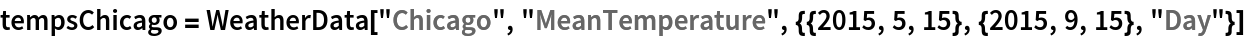Out=In:=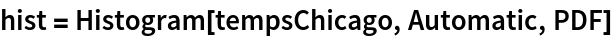Out=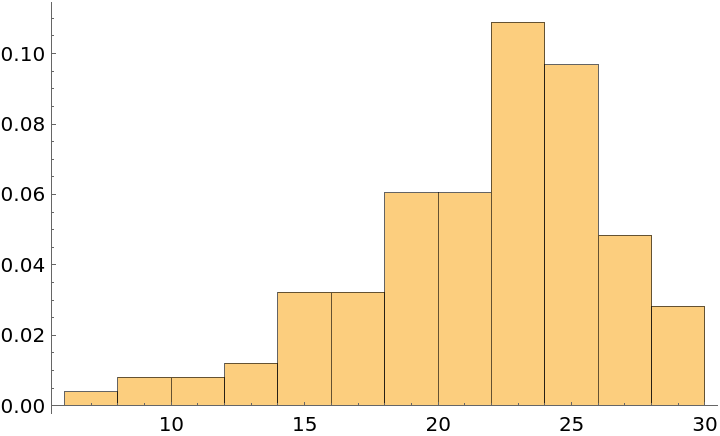Fit the distribution to PERTDistribution:

 In:=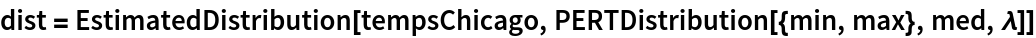Out=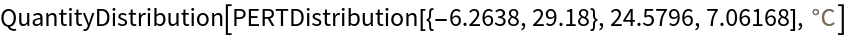Check goodness of fit:

 In:=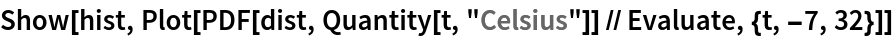Out=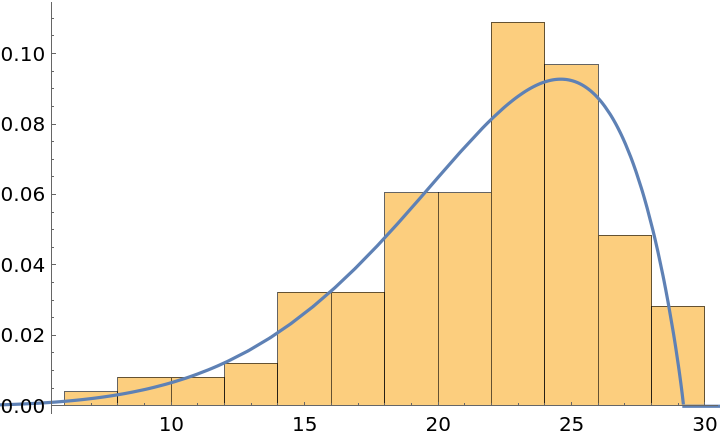Find the estimated distribution for temperature with the SI constants:

 In:=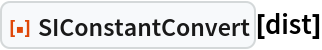Out=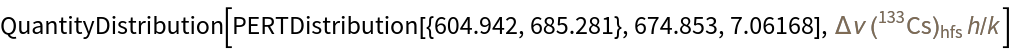Find the mean and standard deviation:

 In:=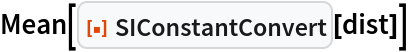Out=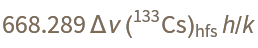In:=Out=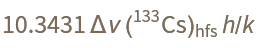Compare the variance and trimmed variance:

 In:=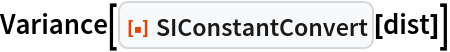Out=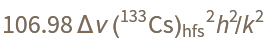In:=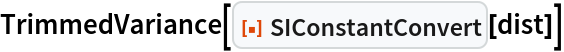Out=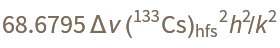Find the first five moments:

 In:=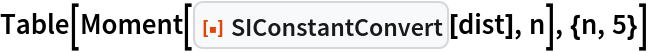Out=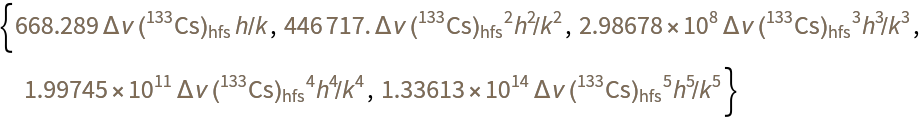### Neat Examples (2)

Convert all instances of physical quantities in the SI Brochure to products of constants:

 In:=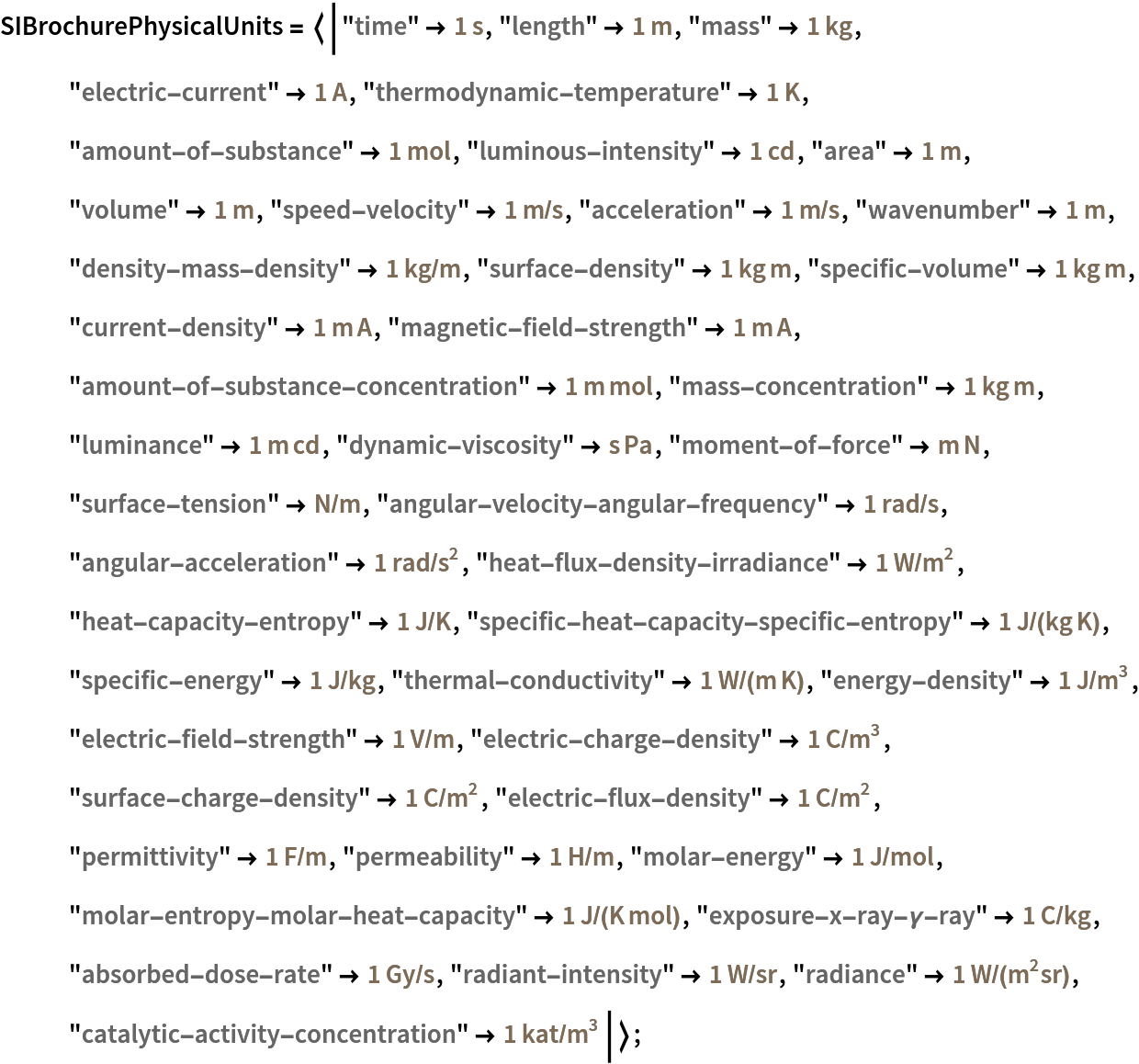In:=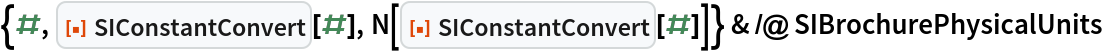Out=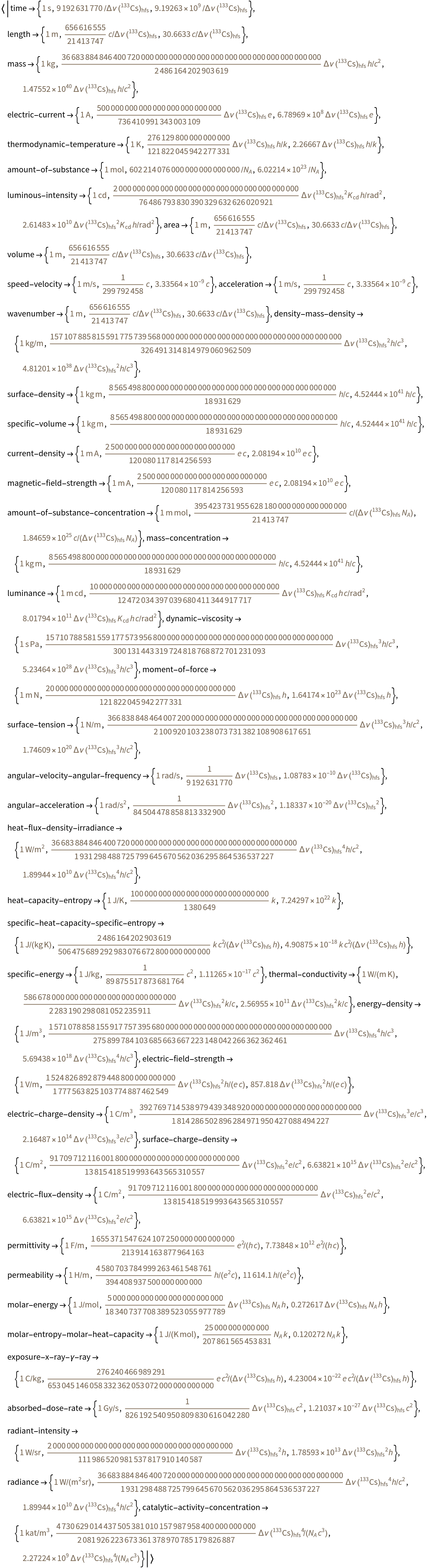Transform units with angles:

 In:=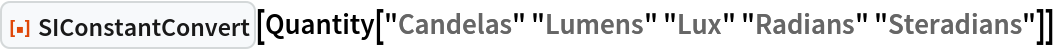Out=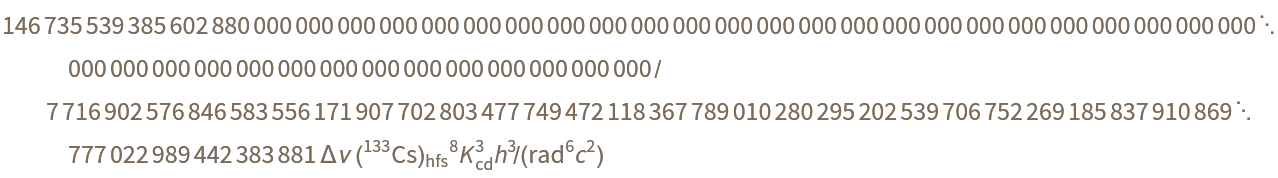Peter Burbery

## Version History

• 1.0.0 – 27 September 2022

## Author Notes

I plan to develop other unit conversion functions for Hartree atomic units for example.